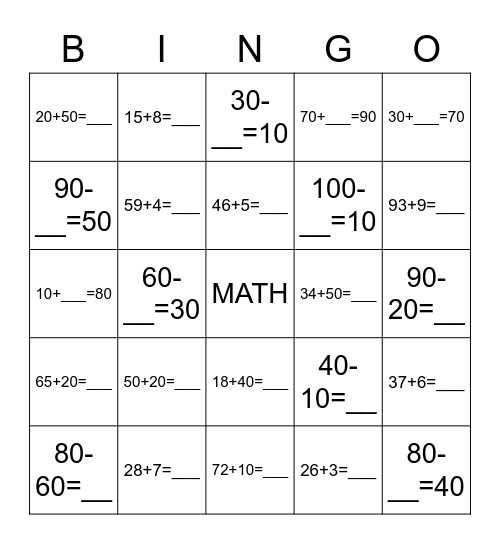# Math BingoThis bingo card has a free space and 24 words: 50+20=___, 28+7=___, 46+5=___, 80-60=__, 30+___=70, 20+50=___, 90-__=50, 15+8=___, 34+50=___, 18+40=___, 100-__=10, 10+___=80, 40-10=__, 37+6=___, 59+4=___, 26+3=___, 90-20=__, 30-__=10, 93+9=___, 60-__=30, 80-__=40, 65+20=___, 70+___=90 and 72+10=___.

## Play Online